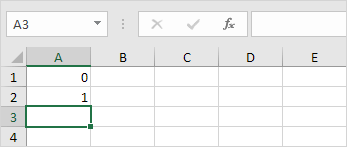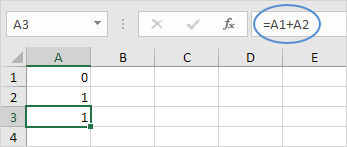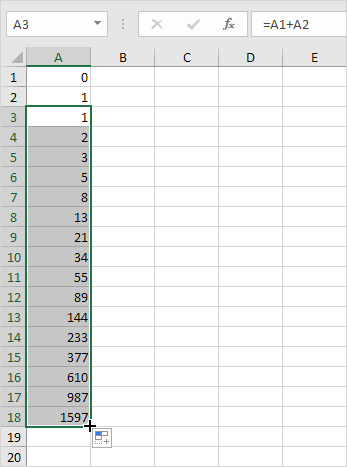# Fibonacci Sequence

It's easy to create all sorts of sequences in Excel. For example, the Fibonacci sequence.

1. The first two numbers in the Fibonacci sequence are 0 and 1.2. Each subsequent number can be found by adding up the two previous numbers.3. Click on the lower right corner of cell A3 and drag it down.

Result. The Fibonacci Sequence in Excel.Go to Next Chapter: Formulas and Functions